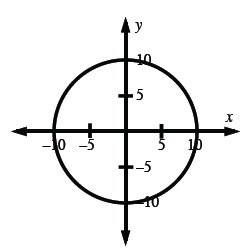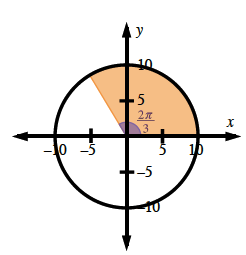### Home > CCA2 > Chapter Ch7 > Lesson 7.1.5 > Problem7-84

7-84.

Sketch a graph of x2 + y2 = 100. Homework Help ✎

1. Is it a function?

2. What are its domain and range?

3. Draw a central angle that measures $\frac { 2 \pi } { 3 }$ radians. If you remove this wedge of the circle, how much area remains?

Does each x-value on the graph of the function have only one corresponding y-value?

Domain: −10 ≤ x ≤ 10
Range: −10 ≤ y ≤ 10See the unit circle graph at right.
What portion of the circle is shaded?

Since $\frac{2\pi}{3}$ is $\frac{1}{3}$ of the total angle in a circle, the remaining area will be $\frac{2}{3}$ of the total area.